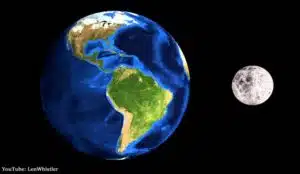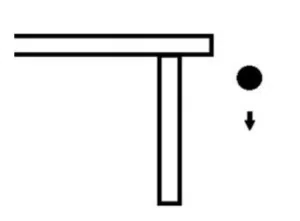# Motion under Gravity

Motion under gravity refers to the movement of an object whose vertical motion is affected by the presence of gravity. The force that attracts objects downwards is GRAVITY. In fact, gravity works towards the centre of the Earth. The mass of the object and the gravitational constant determines the magnitude of the gravitational force. Recall that

WEIGHT = MASS multiplied by GRAVITY.

Mathematically speaking if weight=W, mass=m and gravity=g then:

W=mg.

Weight is a force and is measured in Newtons or kg m/s/s – see Forces & Newton’s First Law. Mass is measured in kilograms (kg) and gravity is an acceleration (m/s/s). For objects reasonably close to the Earth’s surface, gravity is approximated as 9.8 m/s/s (in some questions it may say use g=10 m/s/s).  In the absence of air resistance, like in a vacuum, all objects will fall to the ground at the same rate regardless of their mass, shape and size.Note that weight is different on other planets or celestial objects. Consider the moon, for example. The moon is much smaller in size than the Earth and the gravitational constant is approximately 1.62 m/s/s. As a result, objects weight roughly 17% of what they do on Earth (see more on Gravitation of the Moon). This is why you often see footage of astronauts slowly bouncing over the moon’s surface – gravity is less forceful and the astronauts are lighter there.

Unlike weight, an object’s mass is always the same.

## An example of Motion under GravityConsider a ball that rolls off the edge of a table and falls for 0.5 seconds before it hits the floor. We can find the velocity of the ball when it hits the ground. The ball starts with zero velocity i.e. 0 m/s in the vertical direction. Assuming that gravity is constant and there is no air resistance, the ball will accelerate downwards at a rate of 9.8 m/s/s. If the ball accelerates this way for 0.5 seconds then the final downward speed of the ball will be

$9.8\times 0.5=4.9$ m/s

Note that this is essentially use of the first SUVAT equation. If gravity is constant, any of the SUVAT equations can be used to solve for motion under gravity.

## Motion under Gravity Examples

Calculate the height of the table in the above example. How tall would the table have to be for the ball to have a speed of 7 m/s when it hits the floor and for how long will it fall?

Note 1: An object with motion under gravity does not have a final velocity of 0 when it hits another object.

Note 2: Choose whether up is positive or negative and stick to it. If up is positive, gravitational acceleration will be g=-9.8.

An object is propelled upwards with an initial speed of 100km/hr from a point O.

1. Find the maximum height reached above the point O.
2. The times when the object is 5 metres above the point O.